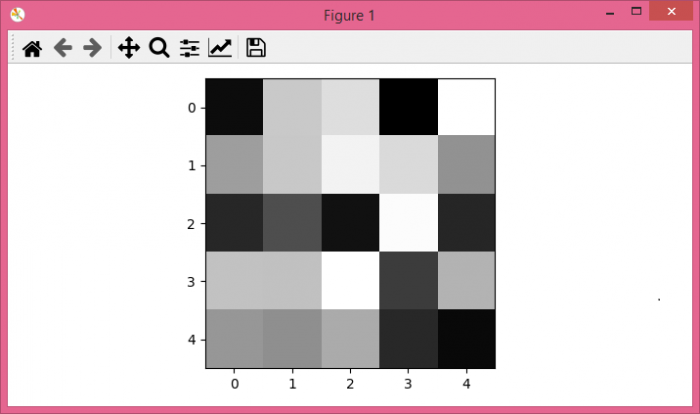# How to show numpy 2D array as grayscale image in Jupyter Notebook?

To show a 2D array as a grayscale image in Jupyter Notebook, we can take the following steps

## Steps

• Set the figure size and adjust the padding between and around the subplots.

• Create a random data using numpy.

• Display the data as an image, i.e., on a 2D regular raster, with gray colormap.

• To display the figure, use Show() method.

## Example

from matplotlib import pyplot as plt
import numpy as np

# Set the figure size
plt.rcParams["figure.figsize"] = [7.00, 3.50]
plt.rcParams["figure.autolayout"] = True

# Random data points
data = np.random.rand(5, 5)

# Plot the data using imshow with gray colormap
plt.imshow(data, cmap='gray')

# Display the plot
plt.show()

## Output

It will produce the following output −